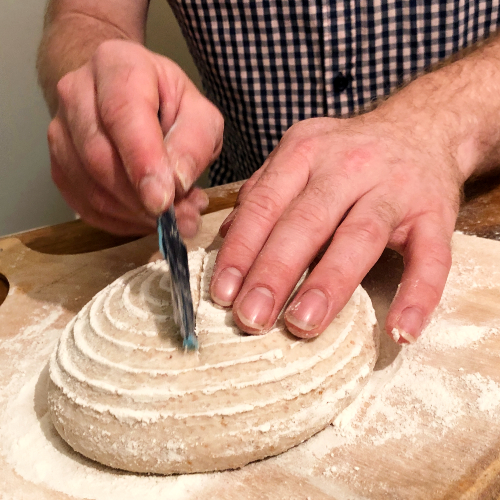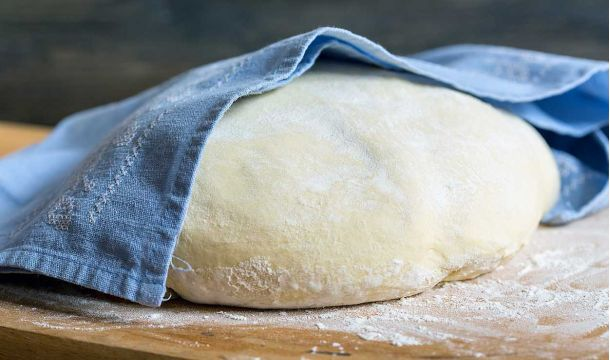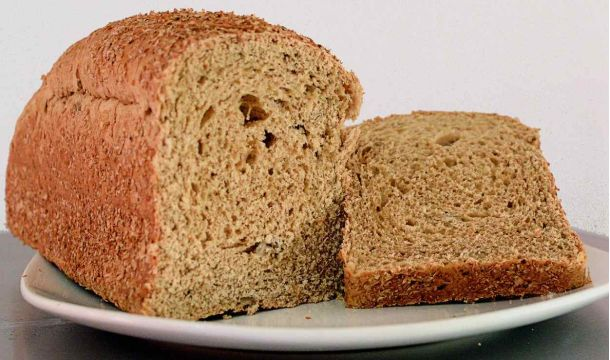# Bakers Percentages & Bakers Formula | All you need to know

Published on
17 May 2020Gareth Busby

Baker’s percentages are challenging to get your head around at first. I know many bakers struggle to understand the point of using percentages and how to use them. But once you’ve got it, you’ll realise they are pretty easy and vital to scale up or down a bread recipe. So enjoy this guide on baker’s percentages and formulas. It’s a top read!

## Baker’s percentage definition

Baker’s percentages (often referred to as “Baker’s math”) display each ingredient in a bread recipe as a percentage based on the total amount of flour. The total flour used in a recipe will always be 100%, and the ingredients are listed as percentages to produce a baker’s formula. Not restricted to bread, baker’s percentages can also be used to prepare cakes and other baked goods.

## Why use baker’s percentages?

To double a recipe provided in grams, ounces, or cups is pretty simple. You just double the ingredients. But what if you wanted to change the amount of dough made by 10% to fit your bread tin better or make bigger or smaller batch sizes? This is where baker’s percentages are useful. As baker’s percentages are based on the weight of the flour required, these things are easier to work out.

Baker’s percentages also make adjusting recipes much more manageable. For example, you might want to lower the water by 3% when using a particular flour to avoid a sticky dough. It’s much easier to have a baker’s formula at hand to do this calculation than to work it out in your head!

Using a baker’s formula ensures that no matter the size of the dough, the ratio of ingredients is always the same. This means that any recipe can be scaled up or down with exact precision. Thus bakers’ percentages are absolutely vital to baking bread commercially.Grab Your Free 7-Day Bread Baking Course!!

Jump onboard to discover the 7 things you are probably doing wrong when you make bread. This 5 minutes a day course teaches you the key principles of bread making so you can make better bread!

To simplify the advantages of using bakers percentages in a list:

• Share recipes easily
• Downsize commercial bread recipes to use at home
• Adapt the number of ingredients in an existing recipe to suit your environment
• Add or remove extra ingredients
• Increase or decrease the batch size of the recipe
• Assess a new recipe to foresee any potential issues

## What do I need to use baker’s percentages?

It’s best to calculate baker’s percentages by weight in grams as the metric system is much easier to calculate. It is possible to use imperial however you will have to do all your calculating in one denomination. You won’t be able to use pounds and ounces.

It is not advised to use cups and spoons to measure your ingredients. It’s not accurate, and when using a baker’s formula, you can only use one measurement. So if you don’t fancy measuring your flour with a teaspoon, you’ll need to use scales!

If you don’t have a good set of scales, this set from My-weigh are fantastic. They are durable, hard-working, accurate and affordable. I’d go for them every time!

For ultimate precession, you may like to get yourself a set of jewellery scales for measuring smaller quantities. These scales measure at 0.01 of a gram accurately and don’t cost much. Using accurate scales like these makes it easy to scale down larger recipes with precision.

Apart from scales, you’ll need a notepad and (probably) a calculator to work out the calculations. I prefer to use a spreadsheet to build a formulate my recipes. Here is a link to a blank baker’s formula spreadsheet, which you can import into Google Sheets or Excel for your own baker’s formula.

## What does a baker’s formula look like?

In a “professional” recipe, individual ingredients are presented as percentages in a baker’s formula. Each ingredient is calculated when making the recipe by multiplying its determined percentage by the total flour weight.

### White tin loaf

`You can see that my tin loaf recipe produces 961 grams of dough. We need 950 grams of dough to fill a 2lb loaf tin, so we make just over to make up for any dough lost in the production process.`

Then if we want to make 4 bread rolls alongside our bread roll, each weighing 80 grams we can increase the size of the recipe:

1 loaf: 1 x 950 = 950

4 rolls: 4 x 80 = 320

Dough required: 950 + 320 = 1270

So to calculate the weights of ingredients in this recipe manually to get the same results as the table above:

For the case of the water:

65 x 760 = 494

494 grams of water

The salt percentage for this bread dough is 2%, so:

2 x 760 = 15.2

15.2 grams of salt

For 1.6% of yeast:

1.6 x 760 = 12.2

12.2 grams of yeast

And so on..!

## How to calculate bakers percentages of ingredients

If we don’t know the baker’s percentage of a recipe, we could work it out with a simple calculation:

Here’s how it works in the white tin loaf example:

First, we’ll calculate the water which is 370 grams into a bakers percentage:

Total flour = 570 grams

Water = 370 grams

(370 ÷ 570) x 100 = 0.65

0.65 x 100 = 65

We now know that the baker’s percentage of water in this recipe is 65%.

This method is then repeated for all of the remaining ingredients to produce our bakers formula.

### Should I round the number up or down to remove the decimal place?

Decimal measurements in a bread recipe can look imposing and, for many bakers, unnecessary. You are welcome to round the decimals into whole numbers when weighing your ingredients. I don’t usually do this as after rounding the decimals from the original recipe, the baker’s formula and then again when the batch size is increased, the recipe changes.

## How to calculate the amount of flour required for the recipe

To increase our original recipe from 1 loaf to 1 loaf and 4 rolls, I increased the total flour weight. But how did I work out that I needed 760 grams of flour? We could use a spreadsheet (which I’ll come onto later) and keep tapping numbers in until the target dough weight (1270 grams) is reached, or we could use a formula to do this for us.

Knowing that 570 grams of flour produces 961 grams of dough, divide the new target dough weight by the total dough in the previous formula. Instead of 1270 grams, we’ll add 10 grams, as some dough will be lost during preparation.

Target total dough weight ÷ previous total dough weight:

1280 ÷ 961 = 1.321

Rounded, this gives us 1.32

So we now know that we need to make our original recipe 1.32 times larger. Thus the total flour of our bigger batch is:

570 x 1.32 = 752.4

Rounded to 750 grams

## How to use a spreadsheet to speed up the calculation of bakers percentages

By setting up a spreadsheet with the values, you can speed up the time taken to work out the ingredients required. You can download my baker’s formula template and import it into google sheets or Microsoft excel. Change the total flour weight at the top of the sheet (B1), and the rest of the ingredients will self-populate.

## How to calculate bakers percentage when using multiple types of flour

A baker’s percentage uses the total amount of flour. That is all of the flour used in the recipe. So, if you are mixing flours in a recipe, combine their weights to make 100%. For example:

White flour @ 300 grams = 75%

Whole wheat flour @ 100 grams = 25%

75% + 25% = 100%

## How to use a baker’s formula with preferments

In a baker’s formula, the baker’s percentage should separate the ingredients used in the preferment. This means the flour from the preferment is included in the total flour weight. Let’s go through an example recipe:

Preferment:

• 100 grams flour
• 80 grams water
• 1 gram yeast

Dough:

• 400 grams flour
• 300 grams water
• 5 grams yeast
• 10 grams salt

For the total flour weight, add the flour in the preferment and the main dough, so we know what 100% is.

100 + 400 = 500g

500g = 100%

Therefore we can calculate the individual baker’s percentages for both instances of the flour:

100 ÷ 500 = 0.2

20% of the flour is pre-fermented

400 ÷ 500 = 0.8

80% of the flour is for the main dough

Then continue with the other ingredients using the formula:

Divide the weight of the ingredient by the weight of the flour, then multiply by 100.

The baker’s formula for this recipe looks like this:

## How to calculate the percentage of the preferment in the dough?

Calculating the pre-fermented flour (PFF) percentage makes you aware of the amount of the mature preferment or sourdough starter used. This is a handy figure to know as dough with a higher PFF percentage requires shorter bulk fermentation.

To calculate the PFF percentage, divide the amount of flour that is pre-fermented by the total amount of flour used:

For the above example:

(100 ÷ 500) x 100 = PFF

0.2 x 100 = PFF

PFF is 20%

## How to use a baker’s formula with sourdough

You can use a baker’s formula with sourdough recipes. As when using preferments, the water and the flour in the starter should form part of the recipes’ formula. To do this the easy way, look at the ratio that you feed your starter, ignoring the amount of old starter.

For example: If you feed your starter in a 1:1:1 ratio of starter, flour and water, the starter is deemed to be 50% flour and 50% water. If you use 150 grams of starter in your recipe, you should enter in your formula: 75 grams of flour and 75 grams of water.

If you prefer a stiffer starter and use a ratio of 1:4:3, the ratio is 4 parts flour and 3 parts water, or (100/7 * 4) 57% flour and (100/7 * 3) 43% water. For 150 grams of starter, this would be 86 grams flour and 64 grams of water. Here’s what a sourdough recipe would look like:

Sometimes a format like this is used:

Often in professional recipes, the starter is built up, and the refreshments are included in the formula:

It uses the chéf method that takes a mature starter and is “built it up”. Calculating the recipe this way provides more accuracy in terms of hydration, but as some of the started weight will evaporate, it’s not always as accurate as you think. Most home bakers prefer to just weigh their starter which is why I’ve shared this version till the end. You be you!

## If I want to adjust my recipe to use less water, how do I do this?

If you feel you need to reduce or increase the amount of water or any other ingredient in a recipe using a baker’s formula makes it easy.

To prevent a slightly sticky dough, I’ll usually reduce the percentage of water in the formula by 2%. But if you know the weight of the ingredient you want to add or remove, we can remove this accurately. Say we have our recipe:

And we want to remove 15 grams of water from the main inclusion.

(15 ÷ 500) x 100 = 0.03

0.03 x 100 = 3

We need to remove 3% of the water from the original percentage:

60 – 3 = 57

Our water percentage is now 57%

57% of 500 = 285 grams

## Standard baker’s percentages for ingredients

There are common relationships between the ratio of ingredients used as a basis for the majority of bread recipes. Yeast and salt typically have a percentage of 1.8-2% and water tends to be around 65% for a stiff white dough, rising to 75% for artisan or whole wheat bread.

## Ending thoughts on percentages and formulas

Knowing the baker’s percentages of a new recipe helps to predict things like how quickly the dough will rise or how wet and sticky it will be. This knowledge, although not essential, enables you to understand recipes better and grow your baking skills. If you want to learn more about baker’s percentages in action, take a look at the dough hydration article. It explains how to change the water ratio in any bread recipe! Please use the comments section below to ask any questions.

## Frequently asked questions about baker’s percentages

Subscribe
Notify ofInline Feedbacks

## Related Articles### How To Control Temperature When Making Bread

14 September 2022### Can I Make Bread Without Rising Twice?

13 September 2022

### Join The Weekly Bread Baker's Newsletter!

Join my weekly baking newsletter to be notified with the latest bread baking tips and trends.

#### Disclaimer

As an Amazon Associate I earn from qualifying purchases. I also earn a commission through other affiliate programs on this site.